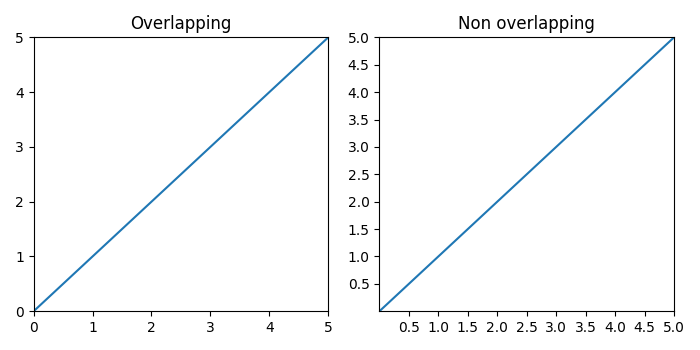# Overlapping Y-axis tick label and X-axis tick label in Matplotlib

To reduce the chances of overlapping between and tick labels in matplotlib, we can take the following steps −

• Create and data points using numpy.

• Add a subplot to the current figure at index 1 (nrows=1 and ncols=2).

• Set and margins to 0.

• Plot and data points and add a title to this subplot, i.e., "Overlapping".

• Add a subplot to the current figure at index 2 (nrows=1 and ncols=2).

• Set and margins to 0.

• Plot x and y data points and add a title to this subplot, i.e., "Non Overlapping".

• The objective of MaxNLocator and prune ="lower" is that the smallest tick will be removed.

• To display the figure, use show() method.

## Example

import matplotlib.pyplot as plt
from matplotlib.ticker import MaxNLocator
import numpy as np
plt.rcParams["figure.figsize"] = [7.00, 3.50]
plt.rcParams["figure.autolayout"] = True
xs = np.linspace(0, 5, 10)
ys = np.linspace(0, 5, 10)
plt.subplot(121)
plt.margins(x=0, y=0)
plt.plot(xs, ys)
plt.title("Overlapping")
plt.subplot(122)
plt.margins(x=0, y=0)
plt.plot(xs, ys)
plt.title("Non overlapping")
plt.gca().xaxis.set_major_locator(MaxNLocator(prune='lower'))
plt.gca().yaxis.set_major_locator(MaxNLocator(prune='lower'))
plt.show()

## Output